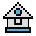Home

# POINT Function

#### Purpose:

To read the color or attribute value of a pixel from the screen.

#### Syntax:

```POINT(x,y)
POINT(function)```

In the first syntax, x and y are coordinates of the point to be examined.

If the point given is out of range, the value -1 is returned.

See the COLOR and PALETTE statements for valid color and attribute values.

POINT with one argument allows you to retrieve the current graphics coordinates.

POINT(function) returns the value of the current x or y graphics coordinates as follows:

 Function Returns 0 the current physical x coordinate. 1 the current physical y coordinate. 2 the current logical x coordinate if WINDOW is active; otherwise, it returns the current physical x coordinate as in 0 above. 3 the current logical y coordinate if WINDOW is active; otherwise, it returns the current physical y coordinate as in 1 above.

#### Example 1:

```10 SCREEN 1
20 FOR C=0 TO 3
30 PSET (10, 10),C
40 IF POINT(10, 10)<>C THEN PRINT "BROKEN BASIC="
50 NEXT C```

#### Example 2:

The following inverts the current state of a point:

```10 SCREEN 2
20 IF POINT(I, I)<>0 THEN PRESET(I, I) ELSE PSET(I, I)```

#### Example 3:

The following is another way to invert a point:

`20 PSET (I, I), 1-POINT(I, I)`